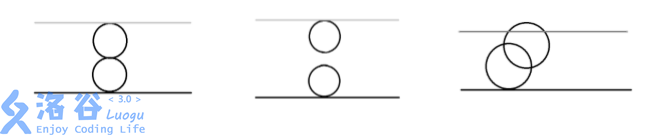# Noip2017奶酪（提高d2t1）

## 检查连通性问题

Posted by yjjr's blog on October 13, 2018

# 题目

## 题目描述

\mathrm{dist}(P_1,P_2)=\sqrt{(x_1-x_2)^2+(y_1-y_2)^2+(z_1-z_2)^2}

## 输出格式

$T$ 行，分别对应 $T$ 组数据的答案，如果在第 $i$ 组数据中，Jerry 能从下 表面跑到上表面，则输出Yes，如果不能，则输出No （均不包含引号）。

## 输入输出样例

### 输入样例#1

3
2 4 1
0 0 1
0 0 3
2 5 1
0 0 1
0 0 4
2 5 2
0 0 2
2 0 4


### 输出样例#1

Yes
No
Yes


## 说明

【输入输出样例 1 说明】【数据规模与约定】

# code

#include<iostream>
#include<cstdio>
#include<cstdlib>
#include<cstring>
#include<cmath>
#include<algorithm>
#define rep(i,a,b) for(int i=a;i<=b;i++)
#define dep(i,a,b) for(int i=a;i>=b;i--)
#define ll long long
#define mem(x,num) memset(x,num,sizeof x)
using namespace std;
inline ll read(){
ll f=1,x=0;char ch=getchar();
while(ch<'0'||ch>'9'){if(ch=='-')f=-1;ch=getchar();}
while(ch>='0'&&ch<='9'){x=x*10+ch-'0';ch=getchar();}
return x*f;
}
const int maxn=2e3+6,inf=0x3f3f3f;
ll cnt=0,T,n,r,h,x[maxn],y[maxn],z[maxn],dis[maxn],Map[maxn][maxn];
int que[maxn],inque[maxn];

void spfa(){
int head=0,tail=1;
que[head]=0,inque=1,dis=1;
while(head<tail){
int now=que[head];
rep(j,0,n+1)
if(Map[now][j]==1&&dis[now]==1&&dis[j]==0){
dis[j]=1;
if(!inque[j])inque[j]=1,que[tail++]=j;
}
inque[now]=0;head++;
}
}
int main(){
T=read();
while(T--){
mem(x,0);mem(y,0);mem(z,0);mem(Map,0);mem(inque,0);mem(dis,0);
n=read(),h=read(),r=read();
rep(i,1,n)x[i]=read(),y[i]=read(),z[i]=read();
rep(i,1,n)
rep(j,1,n){
if(i==j)continue;
double t=sqrt((x[i]-x[j])*(x[i]-x[j])+(y[i]-y[j])*(y[i]-y[j])+(z[i]-z[j])*(z[i]-z[j]));
if(t<=2*r)Map[i][j]=Map[j][i]=1;
}
rep(i,1,n)if(z[i]-r<=0)Map[i]=Map[i]=1;
rep(i,1,n)if(z[i]+r>=h)Map[i][n+1]=Map[n+1][i]=1;
spfa();
if(dis[n+1]==1)printf("Yes\n");else printf("No\n");
}
return 0;
}